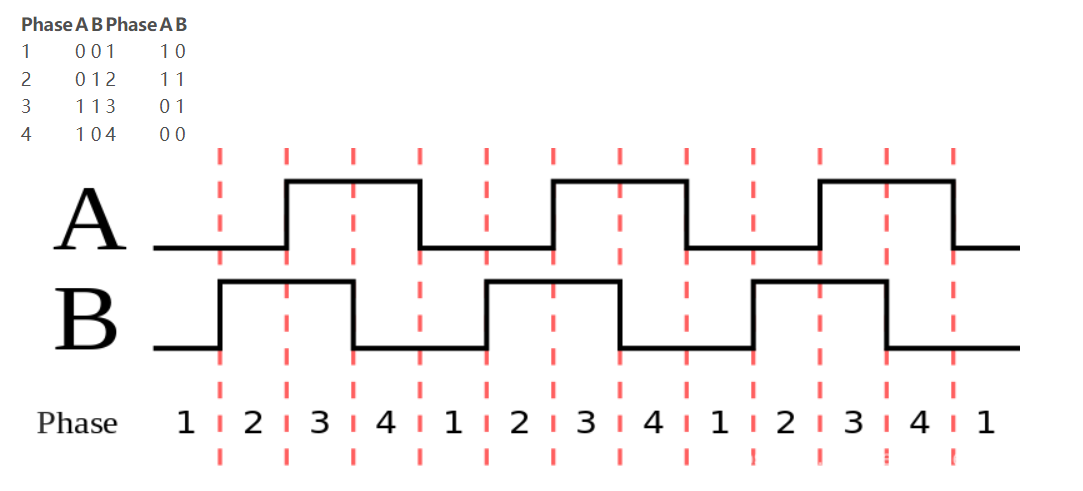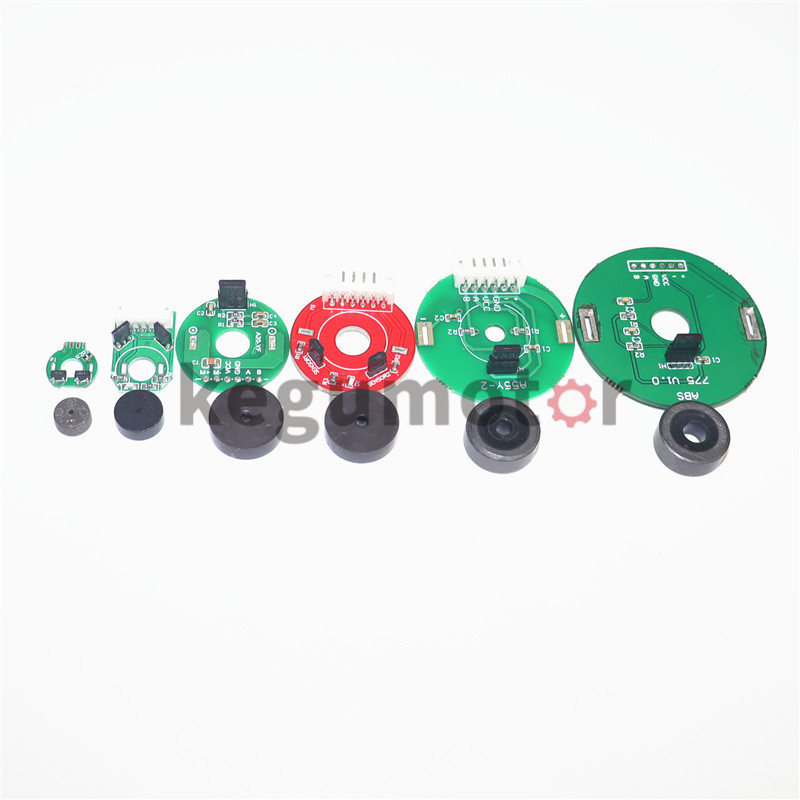Welcome to: Kegu Motor | Micro BLDC Motor|Plastic Planetary Gear Motor|DC Planetary Gearmotor

# Conversion relationship between PPR, CPR and LPR in the encoder

PPR - English abbreviation for the number of pulses per revolution. CPR=PPR*4

Example: A phase 2048 pulses/revolution, B phase 2048 pulses/revolution, the positions of A and B are staggered by a little (called a mathematical 90-degree phase difference), the high pulse is 1, the low is 0, A and B are in each Four combinations of 00, 01, 11, and 10 are formed in one pulse period. This is "4 times frequency", that is, CPR=2048(PPR)x4=8192.CW: Clockwise rotation (Clock Wise) Phase A is output before phase B CCW: Phase B is output before phase A when rotated counterclockwise (Counter Clock Wise)

CPR-parameter description for rotary encoder , refers to the number of pulses (line number) outputted by one rotation. CPR most often stands for counts per revolution, and refers to the number of quadrature decoding states that exist between the two outputs, A and B. When the two outputs A and B are switched between high and low levels, 2 bits of information will appear, representing 4 different states.

The term quadrature decoding describes a method of counting each state change using outputs A and B simultaneously. The result is 4 times the number of counts per pulse or cycle. Therefore, the CPR of the encoder is the PPR of the encoder multiplied by 4.LPR (lines per revolution) is another term similar to PPR.

Lines represent lines etched or printed on the optical encoder disc. Each line on the optical encoder disk represents the output low pulse because they have a one-to-one relationship. Since different manufacturers in the industry have different definitions of resolution, it is necessary to convert the numbers to the same format when comparing products from different encoder manufacturers. Usually PPR=LPR=CPR/4, more information, welcome to communicate at any time.No next

## CATEGORIES